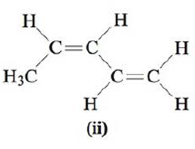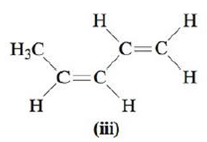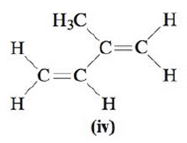Chapter 22, Problem 34E

Chapter
Section
Textbook Problem

# Consider the following four structures.a. Which of these compounds would have the same physical properties (melting point, boiling point, density, and so on)?b. Which of these compounds are trans isomers?c. Which of these compounds do not exhibit cis–trans isomerism?

(a)

Interpretation Introduction

Interpretation: The compounds that would have same physical properties; which are trans isomers and those compounds that do not exhibit cis-trans isomerism are to be stated.

Concept introduction: Structural isomerism occurs when two compounds have same number of atoms but the spatial arrangement of the atoms is different from each other.  These isomers have the same formula but their properties are different. In cis-isomers, the substituents of the double bonded carbons are on the same side of the double bond but in trans isomer, they are on opposite side of double bond.

To determine: The compounds that have same physical properties.

Explanation

Explanation

The compounds that have same physical properties are shown in Figure 1.

The compounds that have same physical properties are,

(b)

Interpretation Introduction

Interpretation: The compounds that would have same physical properties; which are trans isomers and those compounds that do not exhibit cis-trans isomerism are to be stated.

Concept introduction: Structural isomerism occurs when two compounds have same number of atoms but the spatial arrangement of the atoms is different from each other.  These isomers have the same formula but their properties are different. In cis-isomers, the substituents of the double bonded carbons are on the same side of the double bond but in trans isomer, they are on opposite side of double bond.

To determine: The trans isomer among the given compounds.

(c)

Interpretation Introduction

Interpretation: The compounds that would have same physical properties; which are trans isomers and those compounds that do not exhibit cis-trans isomerism are to be stated.

Concept introduction: Structural isomerism occurs when two compounds have same number of atoms but the spatial arrangement of the atoms is different from each other.  These isomers have the same formula but their properties are different. In cis-isomers, the substituents of the double bonded carbons are on the same side of the double bond but in trans isomer, they are on opposite side of double bond.

To determine: The compound that will not undergo cis-trans isomerism.

### Still sussing out bartleby?

Check out a sample textbook solution.

See a sample solution

#### The Solution to Your Study Problems

Bartleby provides explanations to thousands of textbook problems written by our experts, many with advanced degrees!

Get Started

#### Find more solutions based on key concepts